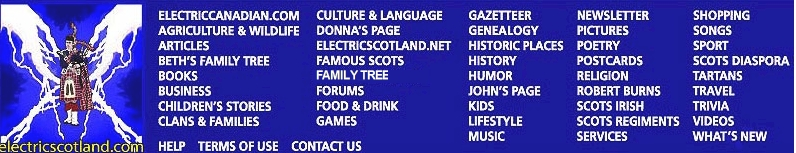Rolfin's Orb Book 2 - Spinal Word Search Puzzle
 `I D E T A U C J T M E L Y G T N R X U Y W R D S Q Q M V A R ` `T X D O B G R Z T K S T E G P C O D W V G O I P U G D B E C ` `O H X M V U I N H Y S D A N O A N S O E V B F A D Z U N L C ` `O Y O K I V A J K Y E R M Z I A M R U R O E D X Q A F Q R H ` `E R G R W Z N Z G N J M I G I P T P U G N R C A M X V D E S ` `A F I K R Q L Q T K Z Q E S U N S H F F R T S L Q I H H Q D ` `R W A X A I A R A G D L H Q Z T S V T R F E R J Q K F V D I ` `K I R K B R R S I V J V O Y X Q B T R Z G S F O I O Y N U K ` `X N I P A H I Q M X K M R D J W Z H A O X L V Q N J A R C B ` `B N L F O K C Z B Y M J F A O T O E O G P Q S J U U J P U U ` `A R A W T F H L U S D Q N J J Z W V Z T D M Q W R J V A J H ` `I W V Z J W R D A X A N A T L F E N X W E R E F B N K M R W ` `F T H A W X V T Y Y A T O V G O S S Q U F E P Z N T S H W Z ` `L A Z A K J T P J B O C D P F Z I D K W L W J W Y C Q F S N ` `L Y H U R I H N E F T V B V C W R V T I F R B Y B N W A R D ` `S P R F P Q T L U A S L L R J O Q O R W M D U N V H W V M Z ` `F U X A T M L F H I E I Y R R F J J T M F W N U O K H L B Q ` `R M N R Q A D M Q R R Z V N O A M Q S J K H V D Z A F F E N ` `B Z O J P N X N Y Y R I A Z Z I P O M A G N U S N I F F U P ` `D L L J A F F J L R K C E W Z L K J G U F U T E Y I E G J H ` `L K F L B T Q U W I E Y L O L L G F G T B S D K X J E O Q S ` `S F E A V T G W N P S B O H Q J O R M C Q X S U V D C X Z Z ` `S C Y P M L B G M I M O H B C M R X T B F N N D F G N B A J ` `I C U L K X M V G K Y T Q B Q Y X G O W B Z S O T Y E N X M ` `U D O C F Y Z Z M Q U A G X J J K I V B Z T H Q V Q I P R D ` `I P Y L S N M M D O S X J H I U Z W N O T W F Y J U G S S Z ` `E N Z P I G X L T N X B F M O V Y N W V J C V B M U A A J W ` `Y B Z W F N L I S P C K R G J Y D Q D F B E C W X N J G D X ` `K B I T W X C P E O X I X Y J J S W R M M R H P K I Y X X R ` `S F L O R A L D Q L H H G L U I S K Y Y X A T X Y I Z V S V ` `ANIKA` `ANNABELLA` `COLIN` `CRIANLARICH` `FAIRY` `FERGUSON` `FLORA` `HEIMAEY` `ICELAND` `JESSE` `MAGNUS` `PIZZA` `POND` `PUFFIN` `REYKJAVIK` `ROBERT` `SPINEL` `THORRI` `TROLL` `VIKING`